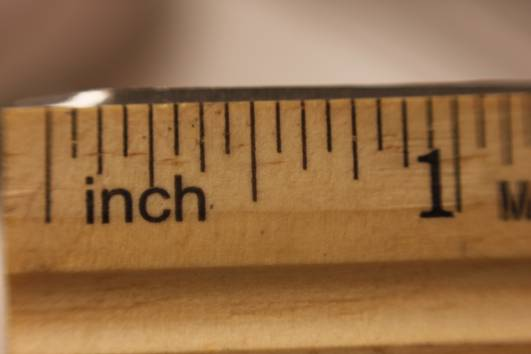How to measure 1/3 inch on ruler?

Truth be told, it's really difficult to measure 1/3 of an inch on a ruler precisely, but there is a way to do it relatively accurately.  Before we get to that, though, let's have a quick look at why it's so difficult to do.Why can't you measure 1/3 of an inch easily on a ruler?

Standard rulers and tape measures divide inches by even measurements.  For example, you are usually able to see a marking for each 1/16 or 1/8 of an inch.  Therefore, you can use a ruler to easily mark a variety of inch divisions, including 1/4, 1/2, 1/8 and 1/16 (and loads more).

The difficulty with measuring 1/3 on a ruler or tape measure is that it isn't a even number, and therefore, it's simply not listed or marked.  You may be able to buy specially designed rulers to help you with this problem, but unless you're needing your measurement to be extremely accurate, there is a way to measure 1/2 of an inch on a ruler.

I'll provide details of how to measure a 1/3 of an inch on rulers that measure in 1/16 gaps, and also rulers that measure in 1/8 gaps.

1/16 rulers

On a ruler that has markings in increments of 1/16, you'll find 1/3 of an inch roughly between the markings of 5/16 and 6/16.  Here's how to work it out:

Take the total increments, which in this case is 16, and divide by three.  That gives you the answer of 5.33, which is roughly halfway between 5/16 and 6/16.  It's not totally accurate, but will serve the purpose for most tasks.

1/8 rulers

The sum is the same as the one we used for the 1/16 ruler, only this time, we divide 8 by 3.  That gives us an answer of 2.66.  What this means is that 1/3 of an inch is located approximately between 2/8 and 3/8.

thanked the writer.